# 2个点旋转角度 unity3d

## unity3D中物体实现任意角度自旋转

2015-05-19 13:40:34 yangyisen0713 阅读数 10669

Input.GetAxis(“Mouse x”) 可取得鼠标横向（x轴）移动增量

Input.GetAxis(“Mouse y”) 可取得鼠标竖向（y轴）移动增量```using UnityEngine;
using System.Collections;

public class NewBehaviourScript : MonoBehaviour {

private bool onDrag = false;  //是否被拖拽//
public float speed = 6f;   //旋转速度//
private float tempSpeed;   //阻尼速度//
private float axisX = 1;
//鼠标沿水平方向移动的增量//
private float axisY = 1;    //鼠标沿竖直方向移动的增量//
private float cXY;
void OnMouseDown()
{
//接受鼠标按下的事件//

axisX = 0f; axisY = 0f;
}
void OnMouseDrag()     //鼠标拖拽时的操作//
{

onDrag = true;
axisX = -Input.GetAxis("moveX");
//获得鼠标增量//
axisY = Input.GetAxis("moveY");
cXY = Mathf.Sqrt(axisX * axisX + axisY * axisY); //计算鼠标移动的长度//
if (cXY == 0f) { cXY = 1f; }

}
float Rigid()      //计算阻尼速度//
{
if (onDrag)
{
tempSpeed = speed;
}
else
{
if (tempSpeed > 0)
{
tempSpeed -= speed * 2 * Time.deltaTime / cXY; //通过除以鼠标移动长度实现拖拽越长速度减缓越慢//
}
else {
tempSpeed = 0;
}
}
return tempSpeed;
}

void Update()
{
// this.transform.Rotate(new Vector3(axisY, axisX, 0) * Rigid(), Space.World); //这个是是按照之前方向一直慢速旋转
if (!Input.GetMouseButton(0))
{
onDrag = false;
this.transform.Rotate(new Vector3(axisY, axisX, 0)*0.5f, Space.World);
}
}
}
```2个点旋转角度 unity3d 相关内容

## [Unity] Unity 3D 中的旋转

2018-05-11 14:24:39 u011232064 阅读数 21462

# Unity 3D 中的旋转

## 一、Unity 3D 中 Rotation

``transform.Rotate(xAngle, yAngle, zAngle);``

1. 上述的x轴、y轴、z轴指的是哪组基？是世界坐标系下的xyz轴，还是局部坐标系下的xyz轴？还是其他？
2. 旋转的正方向如何？
3. 旋转的顺序如何？

## 二、旋转轴

### 1. 旋转轴：Inspector 中 Transform 的旋转数值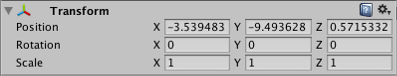The position, rotation and scale values of a Transform are measured relative to the Transform’s parent. If the Transform has no parent, the properties are measured in world space.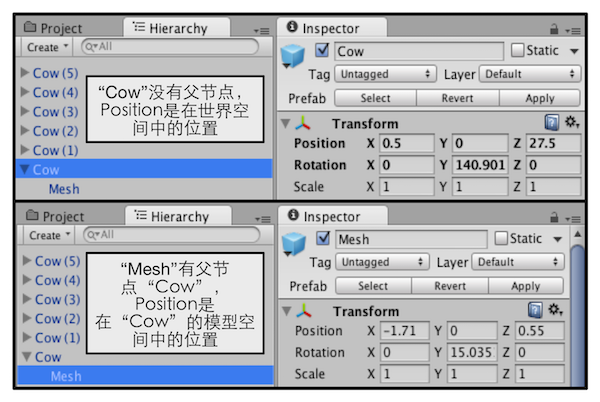### 2. 旋转轴：在Script中使用Rotate函数，在Space.Self中旋转

``````public void Rotate(Vector3 eulerAngles, Space relativeTo = Space.Self);
public void Rotate(float xAngle, float yAngle, float zAngle, Space relativeTo = Space.Self);
public void Rotate(Vector3 axis, float angle, Space relativeTo = Space.Self);``````

``````using UnityEngine;
using System.Collections;

public class Rotate : MonoBehaviour {
public Space m_RotateSpace;
public float m_RotateSpeed = 20f;

// Update is called once per frame
void Update()
{
transform.Rotate(Vector3.up * m_RotateSpeed * Time.deltaTime, m_RotateSpace);
}
}``````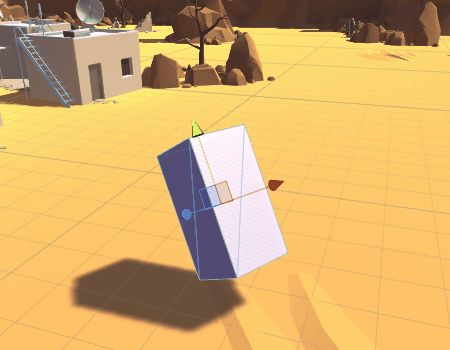### 3. 旋转轴：在Script中使用Rotate函数，在Space.World中旋转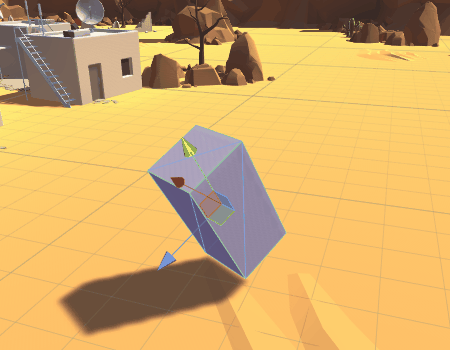## 四、旋转的顺序

### 1. 静态欧拉角

• 情形一：首先绕世界坐标系的x轴旋转90度，再绕世界坐标系的y轴旋转90度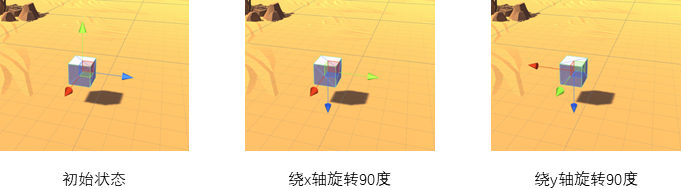• 情形二：首先绕世界坐标系的y轴旋转90度，再绕世界坐标系的x轴旋转90度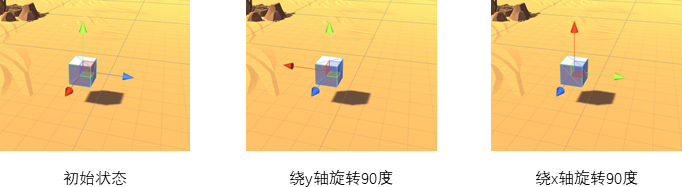### 2. 动态欧拉角

• 情形一：以状态A时的y轴旋转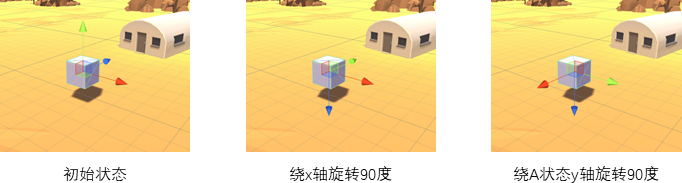• 情形二：以状态B时的y轴旋转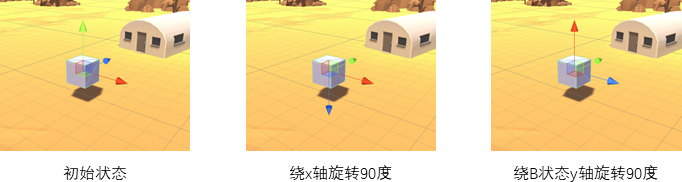Unity中的情况究竟如何呢？直接运行下面的代码会看到结果：

``````void Start () {
transform.Rotate(90, 90, 0, Space.Self);
}``````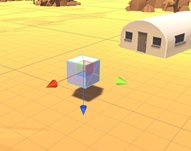``````void Start () {
transform.Rotate(90, 0, 0, Space.Self);
transform.Rotate(0, 90, 0, Space.Self);
}``````

### 3. 静态欧拉角和动态欧拉角的等价形式

Rb == Ry，由定义相同可知。
Ra = (Rb~) * Rx * Rb，要得到绕坐标系E在绕Y轴旋转b后的新坐标系E’下的X轴旋转a的旋转矩阵Ra，先应用Rb~旋转到坐标系E下，然后绕坐标系E下的X轴旋转a，最后应用Rb转回到坐标系E’。
Rc = ((Rb * Ra)~) * Rz * (Rb * Ra)，理由同上。

= Rb * Ra * ((Rb * Ra)~) * Rz * (Rb * Ra)
= Rz * Rb * Ra
= Rz * Rb * (Rb~) * Rx * Rb
= Rz * Rx * Rb
= Rz * Rx * Ry = 左边

``````private void RotateStatic(float a, float b, float c)
{
// 静态欧拉角，依次绕着调用Rotate时的局部坐标系的z,x,y轴旋转a,b,c角度
transform.Rotate(a, b, c, Space.Self);
}

private void RotateDynamic(float a, float b, float c)
{
// 动态欧拉角，绕着调用Rotate时的局部坐标系的y轴旋转b角度
transform.Rotate(0, b, 0, Space.Self);
// 动态欧拉角，绕着调用Rotate时的局部坐标系的y轴旋转a角度
transform.Rotate(a, 0, 0, Space.Self);
// 动态欧拉角，绕着调用Rotate时的局部坐标系的y轴旋转c角度
transform.Rotate(0, 0, c, Space.Self);
}``````

## 参考

1. Unity 中的旋转
2. 欧拉角与万向节死锁

2个点旋转角度 unity3d 相关内容

## Unity3D-限制3D物体的旋转角度

2018-08-16 16:19:22 pz789as 阅读数 5495

``````float CheckAngle(float value){
float angle = value - 180;
if (angle > 0){
return angle - 180;
}
return angle + 180;
}``````

``````float angle = 0;
Vector3 axis = Vector3.left;
rotateXAnim.localRotation.ToAngleAxis(out angle, out axis);``````

``````angle = CheckAngle(angle*axis.x);

if (angle > 90){
angle = 90;
rotateXAnim.localEulerAngles = new Vector3(angle, 0, 0);
}else if (angle < -90){
angle = -90;
rotateXAnim.localEulerAngles = new Vector3(angle, 0, 0);
}``````

``````void MapRotating(Vector3 tempPosition){
Vector3 tempAngle = Vector3.zero;
tempAngle.x = tempPosition.y * 15f / scaleTrans.localScale.x;
tempAngle.y = -tempPosition.x * 15f / scaleTrans.localScale.x;
nowRotate += tempAngle;
nowRotate.x = ClampAngle(nowRotate.x, -90, 90);
SetNowRotValue(nowRotate.x, nowRotate.y, true);
}
static float ClampAngle(float angle, float min, float max) {
if (angle < -360){
angle += 360;
}
if (angle > 360){
angle -= 360;
}
return Mathf.Clamp(angle, min, max);
}``````

2个点旋转角度 unity3d 相关内容

## Unity3D-限制物体的旋转角度（3D和UI均可）

2019-01-15 18:29:24 qq_37896049 阅读数 1174

using System.Collections;
using System.Collections.Generic;
using UnityEngine;

public class TTT : MonoBehaviour
{
public float turnSpeed=5;
// Use this for initialization
void Start()
{

}

// Update is called once per frame
void Update()
{
Rotate();
ClampRot(-30,30);
}

void Rotate()
{
if (Input.GetKey(KeyCode.LeftArrow))
{
transform.Rotate(0, 0, turnSpeed * Time.deltaTime);
}
if (Input.GetKey(KeyCode.RightArrow))
{
transform.Rotate(0, 0, -turnSpeed * Time.deltaTime);
}

}

Vector3 v3;
/// <summary>
/// 限制倾斜角度
/// </summary>
void ClampRot(float minZ,float maxZ)
{
v3 = transform.localEulerAngles;

if (transform.localEulerAngles.z > maxZ && transform.localEulerAngles.z <= maxZ+10)//z控制在30一下
{
v3.z = maxZ;
}
else if (transform.localEulerAngles.z > 350+ minZ && transform.localEulerAngles.z < 360+ minZ)
{
v3.z = 360 + minZ;
}
transform.localEulerAngles = v3;
}

}

2个点旋转角度 unity3d 相关内容

## Unity3d实现物体围绕某一点进行旋转

2016-05-03 17:51:19 qiaoquan3 阅读数 46333

1，让一个物体围绕某一点旋转，有几种方法？分别是什么？

(1)绕坐标轴旋转

`public void Rotate (Vector3 eulerAngles, Space relativeTo = Space.Self);`

public void Rotate (float xAngle, float yAngle, float zAngleSpace, relativeTo = Space.Self);

(2)饶某个向量旋转

public void Rotate(Vector3 axis, float angle, Space relativeTo);

（3）绕轴点旋转

`public void RotateAround(Vector3 point, Vector3 axis, float angel);`

2个点旋转角度 unity3d 相关内容